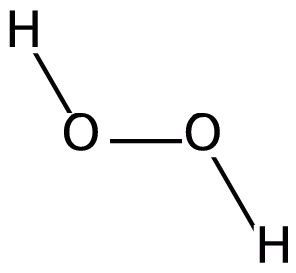## First-Order Reactions

#### Learning Objective

• Design initial rate experiments to determine order of reaction with respect to individual reactants

#### Key Points

• k is the first-order rate constant, which has units of 1/s.
• The method of determining the order of a reaction is known as the method of initial rates.
• The overall order of a reaction is the sum of all the exponents of the concentration terms in the rate equation.

#### Term

• first-order reactionA reaction that depends on the concentration of only one reactant (a unimolecular reaction). Other reactants can be present, but each will be zero-order.

A first-order reaction depends on the concentration of only one reactant. As such, a first-order reaction is sometimes referred to as a unimolecular reaction. While other reactants can be present, each will be zero-order, since the concentrations of these reactants do not affect the rate. Thus, the rate law for an elementary reaction that is first order with respect to a reactant A is given by:

$r = -\frac{d[A]}{dt} = k[A]$

As usual, k is the rate constant, and must have units of concentration/time; in this case it has units of 1/s.Hydrogen peroxideThe decomposition of hydrogen peroxide to form oxygen and hydrogen is a first-order reaction.

## Using the Method of Initial Rates to Determine Reaction Order Experimentally

$2\;N_{2}O_{5}(g)\rightarrow 4\;NO_{2}(g)+O_{2}(g)$

The balanced chemical equation for the decomposition of dinitrogen pentoxide is given above. Since there is only one reactant, the rate law for this reaction has the general form:

$Rate= k[N_{2}O_{5}]^{m}$

In order to determine the overall order of the reaction, we need to determine the value of the exponent m. To do this, we can measure an initial concentration of N2O5 in a flask, and record the rate at which the N2O5 decomposes. We can then run the reaction a second time, but with a different initial concentration of N2O5. We then measure the new rate at which the N2O5 decomposes. By comparing these rates, it is possible for us to find the order of the decomposition reaction.

## Example

Let’s say that at 25 °C, we observe that the rate of decomposition of N2O5 is 1.4×10-3 M/s when the initial concentration of N2O5 is 0.020 M. Then, let’s say that we run the experiment again at the same temperature, but this time we begin with a different concentration of N2O5 , which is 0.010 M. On this second trial, we observe that the rate of decomposition of N2O5 is 7.0×10-4 M/s. We can now set up a ratio of the first rate to the second rate:

$\frac{Rate_1}{Rate_2}=\frac{k[N_2O_5]_{i1}^{m}}{k[N_{2}O_{5}]_{i2}^{m}}$

$\frac{1.4\times 10^{-3}}{7.0\times 10^{-4}}=\frac{k(0.020)^{m}}{k(0.010)^{m}}$

Notice that the left side of the equation is simply equal to 2, and that the rate constants cancel on the right side of the equation. Everything simplifies to:

$2.0=2.0^m$

Clearly, then, m=1, and the decomposition is a first-order reaction.

## Determining the Rate Constant k

Once we have determined the order of the reaction, we can go back and plug in one set of our initial values and solve for k. We find that:

$rate=k[N_2O_5]^1=k[N_2O_5]$

Substituting in our first set of values, we have

$1.4\times 10^{-3}=k(0.020)$

$k=0.070\;s^{-1}$